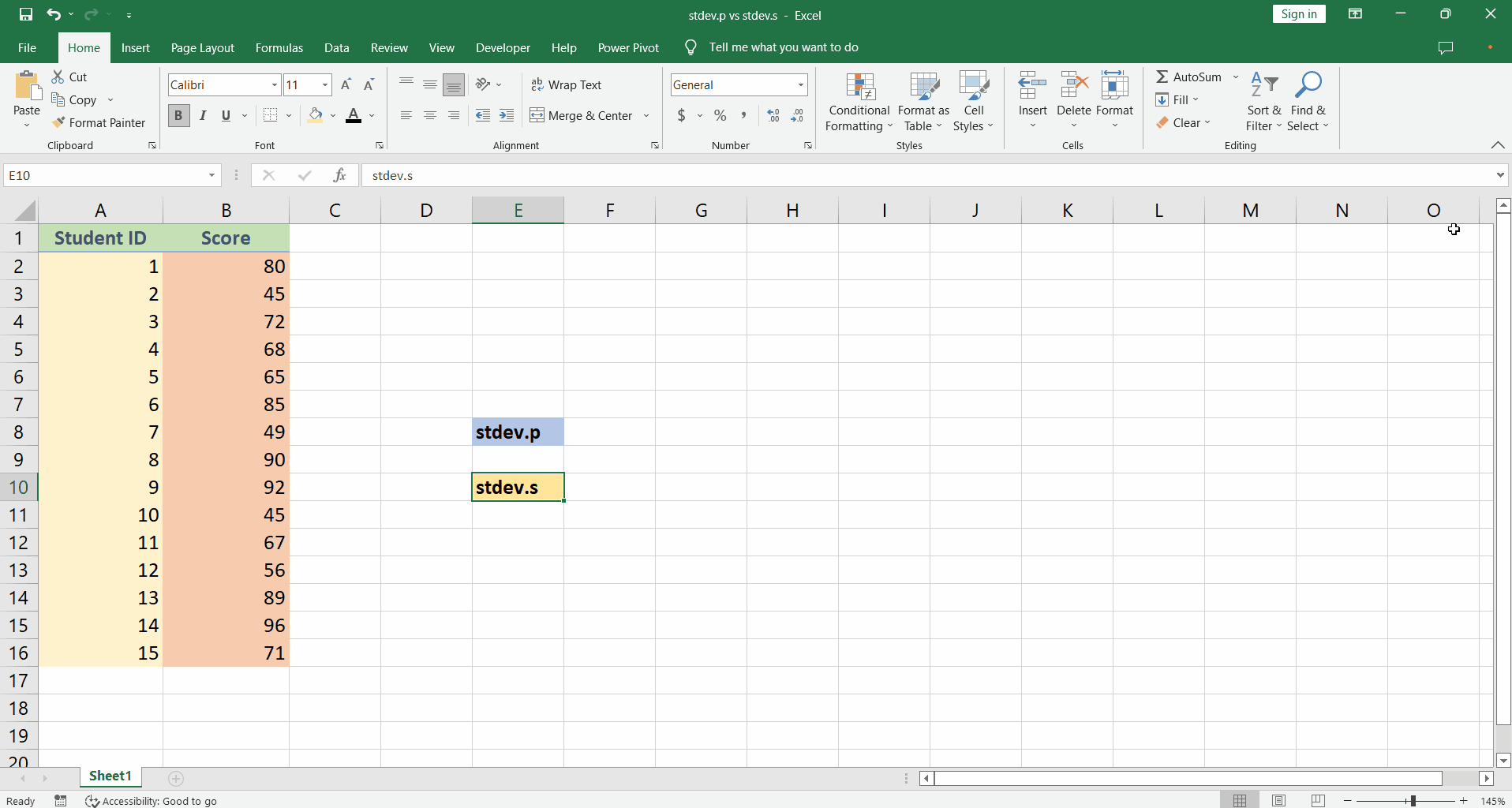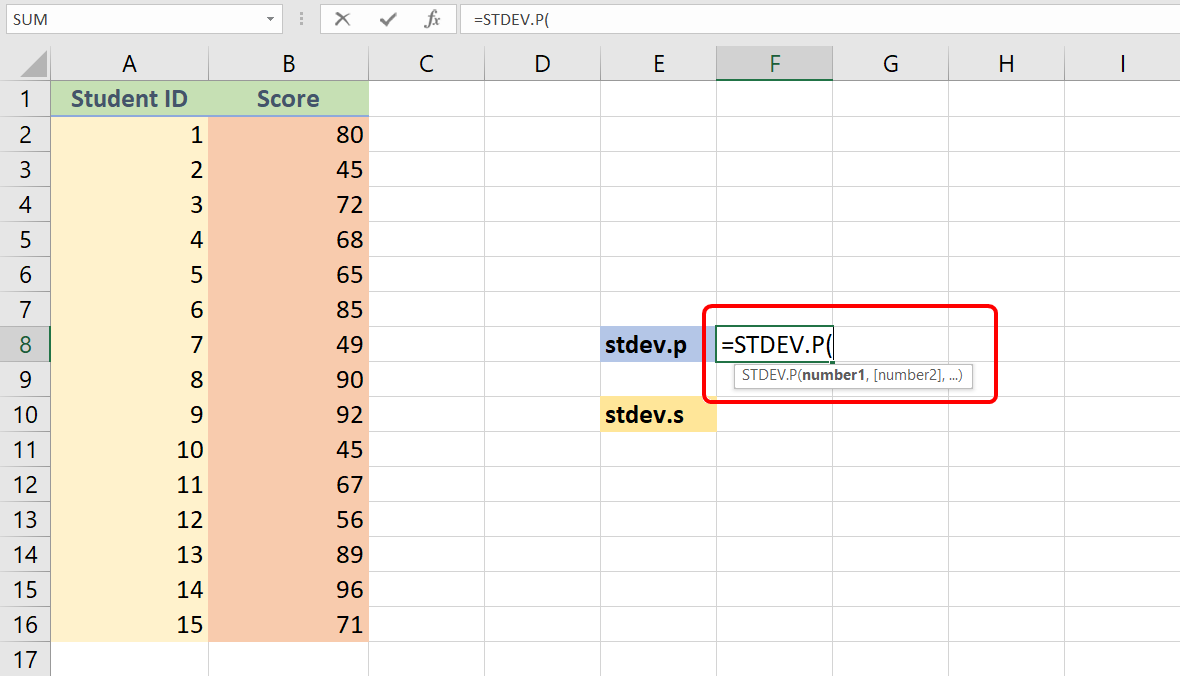# How to use stdev.p and stdev.s functions in Excel

Suppose we have a dataset of student scores in a class.

If this were the total population we will use the the standard deviation formula as follows,

Where the lower case Sigma sign is the standard deviation of the population, the upper case Sigma sign represents the sum, xi is the individual value and is the mean for the population and N is the total population.

However, if it were only a sample from the whole population

Here, xi is the individual value and is the mean for the sample, n is the number of samples and s represents the standard deviation for the sample. So let’s see how we can calculate this in excel.In excel we can calculate the standard deviation of a population as well as that of a sample from a population. So, what is the difference? Let’s learn it in today’s tutorial.

### Step 1 – Understanding syntax– The syntax of both stdev.p and stdev.s functions is similar and they can operate on the numbers separated by comma or the range input from the excel sheet and calculate the standard deviation.
– The syntax is as shown,
=stdev.p(number1, [number2],…)
=stdev.s(number1, [number2],…)
– The numbers in square brackets are optional.
– The formulas can take optional number arguments from 2 up to 255.

### Step 2 – implementing formulas– To implement the formula type equal sign ‘=’ followed by the stdev.p or stdev.s and type the open parenthesis.
– Select the range from B2 to B16 in the given score column and press enter.
– The cell now contains the corresponding functions value.

### When to use STDEV.P and STDEV.S functions

In ideal cases, we would like to find out the standard deviation of a complete data set in a population of interest. However, most of the time the complete data set is not accessible and we get our hands on a very limited sample of the actual data. So with the sample data, we try to find out the approximate standard deviation of the complete data. As a rule of thumb we should:

Use STDEV.P when;

• The dataset consists of all data points from the population of interest.
• The dataset consists of the sample but we don’t want to use the standard deviation of the sample to make an estimate about the complete population.

Use STDEV.S when;

• The dataset contains only a sample from a population of interest and we want to make an estimation about the complete population by using standard deviations. This is what we normally do in our calculations, most of the time, because we don’t have access to the whole data.